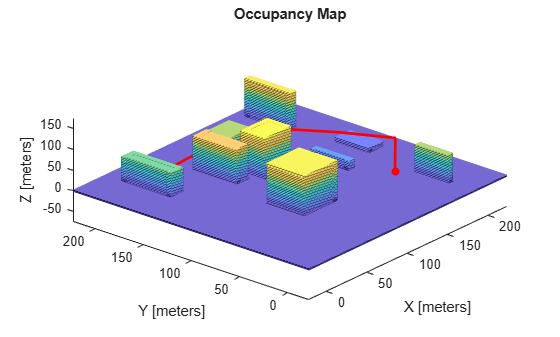# stateSpaceSE3

SE(3) state space

Since R2020b

## Description

The `stateSpaceSE3` object stores parameters and states in the SE(3) state space, which is composed of state vectors represented by [x, y, z, qw, qx, qy, qz]. x, y, and z are Cartesian coordinates. qw, qx, qy, and qz represent the orientation in a quaternion. The object uses Euclidean distance calculation and linear interpolation for the translation component of the state. The object uses quaternion distance calculation and spherical linear interpolation for the rotation component of the state.

## Creation

### Syntax

``space = stateSpaceSE3``
``space = stateSpaceSE3(bounds)``

### Description

````space = stateSpaceSE3` creates an SE(3) state space object with default state bounds for x, y, and z. The state variables qw, qx, qy, and qz corresponding to orientation are not bounded.```

example

````space = stateSpaceSE3(bounds)` creates an SE(3) state space object with state bounds specified as a 7-by-2 matrix. Each row specifies the minimum and maximum value for a dimension of the state in the order x, y, z, qw, qx, qy, and qz. The input `bounds` sets the `StateBounds` property.```

## Properties

expand all

Name of state space, specified as a character vector.

Data Types: `char`

Number of state space dimensions, specified dimensions, returned as a positive integer.

Data Types: `double`

Bounds of state variables, specified as a 7-by-2 matrix of real values.

• The first row specifies the lower and upper bounds of the x state in meters.

• The second row specifies the lower and upper bounds of the y state in meters.

• The third row specifies the lower and upper bounds of the z state in meters.

• The fourth through the seventh rows specify the lower and upper bounds of the state variables qw, qx, qy, and qz respectively, corresponding to orientation as a quaternion.

Note

The `StateBounds` property only affect the Cartesian components of the state. The state variables corresponding to orientation are not bounded.

Example: ```stateSpaceSE3([-10 10; -10 10; -10 10; Inf Inf; Inf Inf; Inf Inf; Inf Inf])```

Example: ```space.StateBounds = [-10 10; -10 10; -10 10; Inf Inf; Inf Inf; Inf Inf; Inf Inf]```

Data Types: `double`

Weight applied to the x, y, and z distance calculation, specified as a positive real scalar. By default, the weight for translation is chosen to be greater than the weight for rotation.

The object calculates distance as:

`$d=\sqrt{{w}_{xyz}\left({d}_{x}^{2}+{d}_{y}^{2}+{d}_{z}^{2}\right)+{w}_{q}{d}_{q}^{2}}$`

,

where wxyz is the weight applied to x, y, and z coordinates, and wq is the weight applied to the orientation in quaternion. dx, dy, and dz are the distances in the x, y, and z directions, respectively. dq is the quaternion distance.

Example: `space.WeightXYZ = 2`

Data Types: `double`

Weight applied to quaternion distance calculation, specified as a positive real scalar. By default, the weight for rotation is chosen to be less than the weight for translation.

The object calculates distance as:

`$d=\sqrt{{w}_{xyz}\left({d}_{x}^{2}+{d}_{y}^{2}+{d}_{z}^{2}\right)+{w}_{q}{d}_{q}^{2}}$`

,

where wxyz is weight applied to x, y, and z coordinates, and wq is the weight applied to the orientation in quaternion. dx, dy, and dz are the distances in the x, y, and z direction, respectively. dq is the quaternion distance.

Example: `space.WeightQuaternion = 0.5`

Data Types: `double`

## Object Functions

 `copy` Create deep copy of state space object `distance` Distance between two states `enforceStateBounds` Reduce state to state bounds `interpolate` Interpolate between states `sampleUniform` Sample state using uniform distribution

## Examples

collapse all

Create a 3-D occupancy map and associated state validator. Plan, validate, and visualize a path through the occupancy map.

Load and Assign Map to State Validator

Load a 3-D occupancy map of a city block into the workspace. Specify a threshold for which cells to consider as obstacle-free.

```mapData = load('dMapCityBlock.mat'); omap = mapData.omap; omap.FreeThreshold = 0.5;```

Inflate the occupancy map to add a buffer zone for safe operation around the obstacles.

`inflate(omap,1)`

Create an SE(3) state space object with bounds for state variables.

```ss = stateSpaceSE3([-20 220; -20 220; -10 100; inf inf; inf inf; inf inf; inf inf]);```

Create a 3-D occupancy map state validator using the created state space.

`sv = validatorOccupancyMap3D(ss);`

Assign the occupancy map to the state validator object. Specify the sampling distance interval.

```sv.Map = omap; sv.ValidationDistance = 0.1;```

Plan and Visualize Path

Create a path planner with increased maximum connection distance. Reduce the maximum number of iterations.

```planner = plannerRRT(ss,sv); planner.MaxConnectionDistance = 50; planner.MaxIterations = 1000;```

Create a user-defined evaluation function for determining whether the path reaches the goal. Specify the probability of choosing the goal state during sampling.

```planner.GoalReachedFcn = @(~,x,y)(norm(x(1:3)-y(1:3))<5); planner.GoalBias = 0.1;```

Set the start and goal states.

```start = [40 180 25 0.7 0.2 0 0.1]; goal = [150 33 35 0.3 0 0.1 0.6];```

Plan a path using the specified start, goal, and planner.

`[pthObj,solnInfo] = plan(planner,start,goal);`

Check that the points of the path are valid states.

`isValid = isStateValid(sv,pthObj.States)`
```isValid = 7x1 logical array 1 1 1 1 1 1 1 ```

Check that the motion between each sequential path state is valid.

```isPathValid = zeros(size(pthObj.States,1)-1,1,'logical'); for i = 1:size(pthObj.States,1)-1 [isPathValid(i),~] = isMotionValid(sv,pthObj.States(i,:),... pthObj.States(i+1,:)); end isPathValid```
```isPathValid = 6x1 logical array 1 1 1 1 1 1 ```

Visualize the results.

```show(omap) hold on scatter3(start(1,1),start(1,2),start(1,3),'g','filled') % draw start state scatter3(goal(1,1),goal(1,2),goal(1,3),'r','filled') % draw goal state plot3(pthObj.States(:,1),pthObj.States(:,2),pthObj.States(:,3),... 'r-','LineWidth',2) % draw path```## Version History

Introduced in R2020b